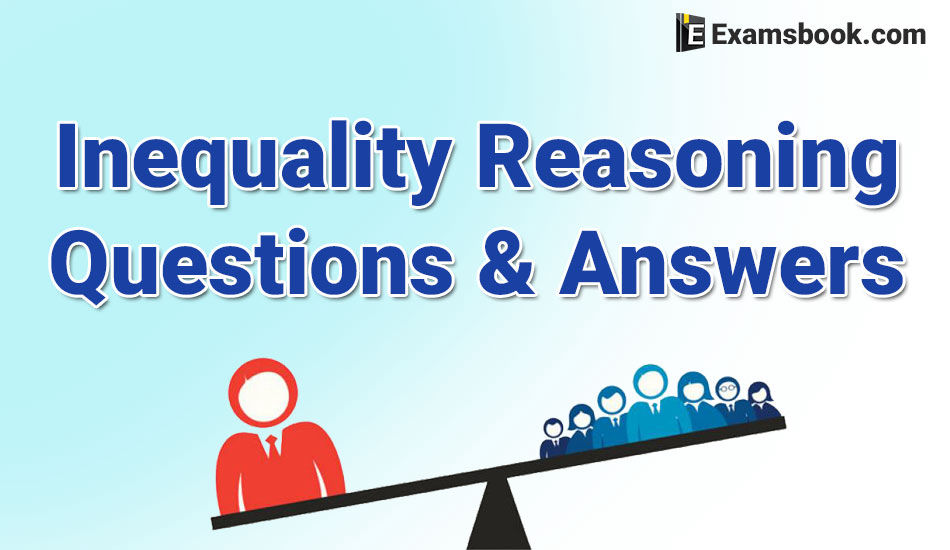• Save

# Inequality Reasoning Questions and Answers for SBI and Bank PO

Vikram SinghLast year 51.9K ViewsMost of the students confused in finding inequality reasoning questions and answers that where they can practice best. They want to prepare important Inequality logical reasoning questions without wasting their time.

So, here you can get the important and selective Inequality reasoning questions and answers for SBI, SSC, Bank exam and other competitive exams. Let's do some practice work.

You can obtain important and selective input and output reasoning questions and answers for SSC, Bank exam and other competitive exams.

## Inequality Reasoning Questions and Answers:

Direction: (1-5): In these question, @, #, $, * & % symbols are used for different meaning as follows: ‘A @ B’ means A is not less than B. ‘A # B’ means A is neither less than nor equal to B. ‘A$ B’ means A is neither less than nor greater than B.

‘A * B’ means A is not greater than B.

‘A % B’ means A is neither greater than nor equal to B.

Considering all the statement in each question true, find that which of the following two conclusion – I & II is definitely true.

Give the answer (A) if only conclusion I is true.

Give the answer (B) if only conclusion II is true.

Give the answer (C) if either conclusion I or conclusion II is true.

Give the answer (D) if neither conclusion I nor conclusion II is true.

Give the answer (E) if conclusion I & conclusion II – both are true.

Q.1. Statement: L * P, P % V, V # D

Conclusion:  I. L * V

II. L $D Ans . D Q.2. Statement: V * W, W$ H, H @ I

Conclusion:  I.  V * I

II. I * W

Ans .  B

Q.3. Statement: N @ W, W # H, H % T

Conclusion: I. H % N

II. T # W

Ans .  A

Q.4. Statement: F # R, H % R, L * H

Conclusion: I. F # L

II. R @ L

Ans .  A

Q.5. Statement: J @ K, K % M, M # T

Conclusion: I. K % T

II. K @ T

Ans .  D

Direction: (6-13) In these questions, symbols @, #, *, $& © are used for different meaning as shown below: ‘A @ B’ means A is not greater than B. ‘A # B’ means A is neither greater than nor equal to B. ‘A*B’ means A is not less than B. ‘A$ B’ means A is neither less than nor equal to B.

‘A © B’ means A is neither greater than nor less than B.

Considering all the statements in each question true, find that which of the following two conclusions – I & II is definitely true.

Q.6. Statement: H $K, K © R, R @ J, J # F Conclusion: I. R # H II. F$ R

III. H $J (A) Only I & II are true. (B) Only I is true. (C) Only II is true. (D) Only II & III are true. (E) All are true. Ans . A Q.7. Statement : L ≤ M, M * P, M # D, D$ F

Conclusion : I. L @ P

II. P ≤ D

III. P > D

(A) Only I is true.

(B) Only II is true.

(C) Either I or III is true.

(D) Only I & II are true.

(E) None of these

Ans .  B

Q.8. Statement:

T @ V, V # Q, Q © L, L * M

Conclusion: I. M @ Q

II. T @ L

III. T # L

(A) Only I is true.

(B) Only II is true.

(C) Only III is true.

(D) Only I & III are true.

(E) None of these

Ans .  D

Ask me in the comment section, if you face any problem while solving inequality logical reasoning questions. Visit next page if you want more inequality logical reasoning questions with Answers.

### Vikram Singh

Providing knowledgable questions of Reasoning and Aptitude for the competitive exams.

## Report Error: Inequality Reasoning Questions and Answers for SBI and Bank PO